Ramanujan’s Sum: 1 + 2 + 3 + 4 + · · · + ∞ = -1/12? Really?

in steemstem •  2 years ago  (edited)

Hey guys, today I bring you a different type of post. So far, I have shared with you some amazing scientific posts, but only discussed the theoretical part in a lucid manner so that everyone can understand the underlying physics of incredible natural phenomena.

But today's post is going to be a mathematical one. We'll discuss about what is the sum of infinite natural numbers and also see the results of different mathematicians. However, I'll try my best to keep the post very much simple, logical and understandable for everybody, even the non-scientific readers. So, let's begin!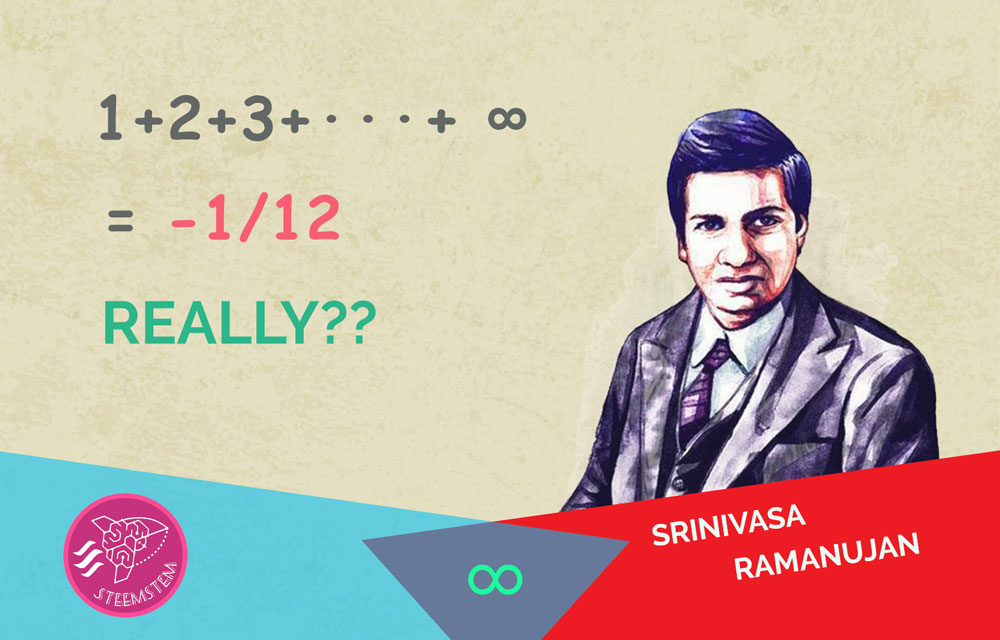"How can finite grasp infinity?".....
John Dryden

The inconsistency and weirdness of infinity makes it more interesting to play with it. I just love math especially arithmetic and infinite number series. Today we are going to discuss the most popular infinite series, 1 + 2 + 3 + 4 + · · · + ∞ and its sum. Results may give your logic a shock, so be prepared. We shall also check other infinite series used to find the summation of the infinite numbers (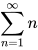)

If you look at the infinite series 1 + 2 + 3 + 4 + · · · you'll find that its sum cannot give a definite value in the general sense, but diverges towards infinity. So this series is called divergent series. Basically, a divergent series is a type of infinite series whose infinite sequence of partial sums does not have a finite limit. Some of you may not be able to grasp what I just said. So, to understand it better let's take a look what a partial sum is?

As the name suggests, a partial sum is the sum of some specific part of the sequence or series. The sum is calculated from the first term to that specific term. To make it more clear take a look at the partial sums of the series 1 + 2 + 3 + 4 + · · ·

1st term (1) = 1
1st term + 2nd term (1+2) = 3
1st term + 2nd term + 3rd term (1+2+3) = 6
1st term + 2nd term + 3rd term + 4th term (1+2+3+4) = 10

So, the resultant partial sums of the series 1 + 2 + 3 + 4 + · · · are 1, 3, 6, 10, 15... etc.

So, now you must have understood what a partial sum is.The partial sums we got may also be called triangular numbers, because they can be arranged as an equilateral triangle

Again the nth partial sum of this special series 1 + 2 + 3 + 4 + · · · is given by this simple formula:

1 + 2 + 3 + 4 + · · · + n =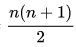So, you can easily see from the formula that the value of the partial sums diverges towards +∞. And hence this is a divergent series.

As we learnt about divergent series, it becomes important to learn also about convergent series. So, what a convergent series is?

Take a look at this series:As you can see, as you move towards the last term of the series, the term becomes smaller and smaller, and we can say that it tends towards zero. Also their partial sums tend to a limit. These type of series are called convergent. We can find a definite sum for these type of series.

Some of the curious minds may have doubt about it: How can a series with infinite number of terms can have a definite value? It should be infinite doesn't it? (Don't worry even the great ancient philosopher, Zeno, was confused with this question.)

No, not actually. The series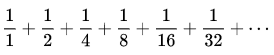converges to '2'. Let me show you how.

Here is the visual representation of the series:Area of first square + Area of second square = (1+1) sq. units = 2 sq. units

As you can see in the figure, we have two squares (each of 1 sq. unit area), one is whole whose area represents the first term of the series i.e. 1, and the second square represents the sum of all the later terms. As you can see we have divided the second square into different parts: Red = 1/2, Blue = 1/4, Yellow = 1/8, Green = 1/6 and so on. So, in a similar way even if we divide the second square into infinite number of parts, the sum of their areas will still be 1 sq. units. And this what the series represent, and so we get the answer '2'.

Again if you are familiar with geometric series (you must have read it in your high school), here is a beautiful formula for calculating infinite geometric series: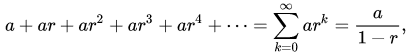when -1 < r < 1
[r is the common ratio and a is the first term, for our series r = 1/2 and a = 1]

So, if we implement the formula in our infinite series, we will get:[clearly -1 < 1/2 <1]

If you are not convinced with this, then there is yet a simpler way to do this:

Take,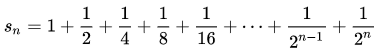Then,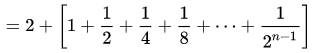Now by subtracting sn from 2sn we get,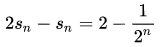, as n approaches infinity sn tends towards '2'.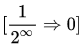Simple, isn't it?

I think we diverted a lot, let's move back to our infinite natural number series, the famous Ramanujan sum.

The Ramanujan’s Sum of Infinite Natural Numbers

Although we know that the sum 1 + 2 + 3 + 4 + · · · is divergent and we cannot find a definite value, yet Ramanujan developed a method to calculate a value for this expression.

Srinivasa Ramanujan was a genius Indian mathematician, who lived during British rule in India. Though he had no formal training in pure mathematics, he made great contributions to mathematical analysis, number theory, infinite series and continued fractions, including solutions to mathematical problems considered to be unsolvable.

Ramanujan developed his own methods to solve these type of infinite series. Ramanujan solved the infinite natural number series in two different ways, the simpler one is as follows:From Ramanujan's original notebook

So, let me write the whole thing again for you: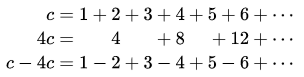As you can see, Ramanujan took the series as a constant ‘c’ and subtracted 4 times of c from c to get a series like this one: 1 + 2 + 3 + 4 + · · ·

But how come he got : 1 - 2 + 3 - 4 + 5 - 6 + · · · =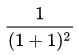Well he knew that,=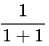and,Really confused, aren’t you? Let’s discuss these two new series one by one.

First take a look at the series 1 – 1 + 1 – 1 + · · · This is also an infinite series and is not convergent. It is also known as Grandi’s series. As it is a divergent series, it lacks a definite sum.

If we put brackets in the series in the following way we’ll get an answer “0” :
(1 - 1) + (1 - 1) + (1 - 1) + · · · = 0 + 0 + 0 + · · · = 0

Again if we put the brackets in little different way leaving the 1st term aside, we’ll get the answer “1” :
1 + (1 - 1) + (1 - 1) + (1 - 1) + · · · = 1 + 0 + 0 + 0 + · · · = 1

What a confusing series! And that's why this is a divergent series. However, mathematicians have one more weird answer for this series i.e. ½. If we treat this series as regular convergent series and use our general algebraic method, we get a special answer ½ for this sum:

S = 1 − 1 + 1 − 1 + · · ·
1 − S = 1 − (1 − 1 + 1 − 1 + · · ·) = 1 − 1 + 1 − 1 + · · · = S
1 − S = S
1 = 2S,
Therefore, S = ½
i.e. 1 − 1 + 1 − 1 + · · · = ½

And there is yet another method to solve this. Remember the infinite geometric series (a + ar + ar2 + ar3 + · · ·) discussed before, whose sum we got by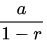where -1 < r < 1. What if we put a=1 and r=-1?

Wait, r cannot be -1, because we have a condition -1 < r < 1. Well, let’s ignore that condition for a moment and see what happens:

We get the series,

1 – 1 + 1 – 1 + · · · ==Maybe this is how Ramanujan got his.

Yet, some smart people may not accept these unrigorous methods. They may say, since the series does not converge it is misleading to speak of its "sum". So for them, there are some more official methods to prove the result. We will get the same ½ if we do Cesáro sum or Abel sum of the Grandi’s series. [Cesáro sum of a series is the average of all of its partial sums.] Another method can be through graphical representation of the Grandi’s series. However, I am not going to discuss the explanation of these methods here, because, the post is turning much longer than I expected and if I start discussing these things then the post will become way more longer and complicated.

So, let’s directly move on to the next series, 1 - 2 + 3 - 4 + · · ·, and see what result does it give. This one is also a divergent infinite series as you can see the partial sums (1, -1, 2, -2…) of this also does not tend towards any finite limit. This series is even more complicated than the previous one and is not solvable even through Cesáro summation. It requires some even more complex summations like Abel summation. Again, I am not going to discuss this complicated method here. Don’t be disheartened, I have some other simpler methods to show this sum. One less rigorous method is as follows:

S   =   1 - 2 + 3 - 4 + 5 - 6 + · · ·
0 + S =   0 + 1 - 2 + 3 - 4 + 5 - · · ·
....................................................
2S   =   1 - 1 + 1 - 1 + 1 - 1 + · · ·      <======= [Grandi's series]

And here we got our Grandi's series and we know its value. So we have,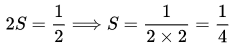Some of you might say that we cannot put ‘0’ at the beginning and add like that. That’s not consistent with mathematical rules. Well for you I have another credible method to show this:

Ramanujan wrote:

1 - 2 + 3 - 4 + · · · =And we know that,=So we can write:= (1 - 1 + 1 - 1 + · · ·)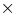(1 - 1 + 1 - 1 + · · ·)

You may think, how can we square this infinite Grandi’s series, isn’t it complicated? Yeah, it is. It will require infinite number of multiplications and additions to find the result. But I am a simple guy and I am not going to do that crazy stuff. I will try to explain this in a much simpler way – by a visual representation of the sum:

We can write,And represent it visually in the following manner: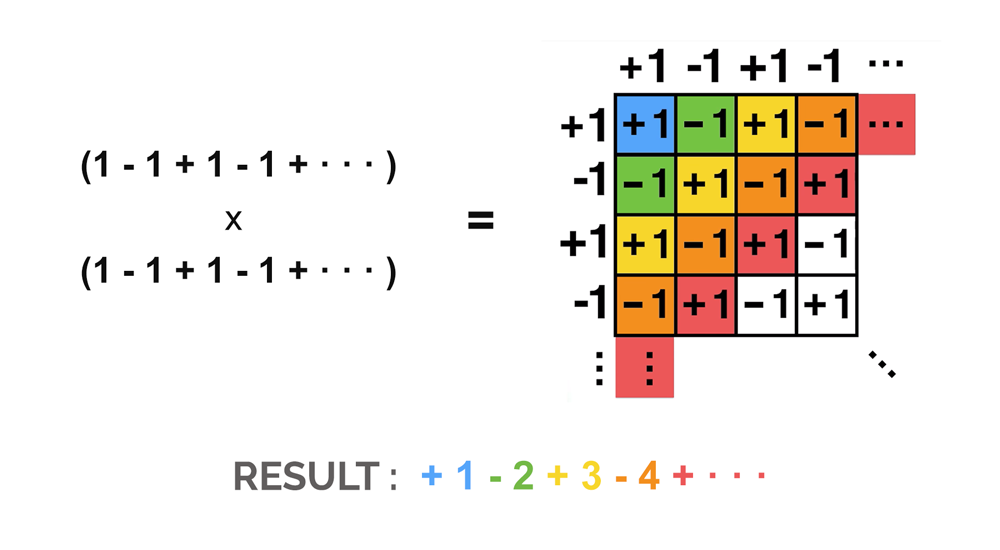If you observe the diagonal shading and add the numbers, you’ll get the following series:
1 - 2 + 3 - 4 + · · ·

And this is how we can write:==After getting all the important results let’s come back to Ramanujan’s sum and his result. Now you can easily understand how he wrote this: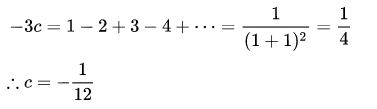Thus, 1 - 2 + 3 - 4 + · · · =So, the seemingly illogical sum gets proved. But…

Is There Something Wrong?Image credits: Freepik

This result may look fascinating, but if you write this answer in your math paper, you may get a big 0!

Generally, it is not considered correct to manipulate infinite series as if they were finite sums. Adding zeroes at arbitrary positions in an infinite divergent series may lead to inconsistencies in the result. For example, the step 4c = 0 + 4 + 0 + 8 + · · · is not justified by the additive identity law. Even adding a single zero at the front of the series (like we did for 1 - 2 + 3 – 4 + · · ·) can also lead to inconsistent results. For example:

If,

1 + 2 + 3 + 4 + · · · = x ....(i)

Adding 0 to the beginning of both sides,

0 + 1 + 2 + 3 + · · · = 0 + x = x ....(ii)

Subtracting equation (ii) from (i) we get,

(1 - 0) + (2 - 1) + (3 - 2) + (4 - 3) + · · · = (x - x)

1 + 1 + 1 + 1 + · · · = 0 ...(iii)

Now adding 0 on both sides again,

0 + 1 + 1 + 1 + 1 + · · · = 0 + 0 ...(iv)

Now again subtracting (iv) from (iii),

1 + 0 + 0 + 0 + · · · = 0

i.e., 1 = 0

Which is contradictory, and thus our process of adding zeros at arbitrary places in an infinite series is not correct.

There is one remedy for this issue. We can restrain the places where zeroes may be inserted and keep track of each term in the series by attaching a dependence on some function. For example, in the series 1 + 2 + 3 + 4 + · · · where each term n is just a number, if we promote n to a function n-s, where ‘s’ is a complex variable, then one can be sure that only like terms are added. The resulting series can be manipulated in a more legal fashion.

Well, there are many such ways to do it, but the results obtained can only be used for some special conditions. We’ll discuss that later in detail, first let’s see what are the methods.

One method can be through analytic continuation of Riemann zeta function (denoted by(s)) also known as zeta function regularization. What is Riemann zeta-function?

Riemann zeta function works for Dirichlet series of the form,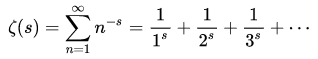In Riemann zeta function variable ‘s’ can be a complex number, however, the real part of s must not be less than or equal to 1. This is because, for the values of the real part of s greater than 1, the Dirichlet series converges but when it is equal to 1 or less it diverges.

But we can get our divergent series 1 + 2 + 3 + 4 + · · · by setting s = -1, which is not allowed. This is where Analytic Continuation comes into play. Analytic continuation is a technique by which we can extend the domain of a given analytic function. Analytic continuation often succeeds in defining further values for a function, for example, it can define new region for an infinite series representation which was initially divergent. [Don't worry I will show it to you visually.]

So, by using analytic continuation of Riemann zeta function (zeta function regularization) we can extend its domain to give the value of 1 + 2 + 3 + 4 + · · · =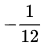. Let's see how:

We know that,Now, by multiplying the function with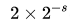we get,And if we carry out a subtraction in the following way we get: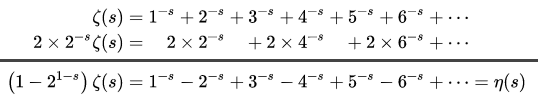Therefore, we haveand it is perfectly okay according to mathematical rules. And by analytic continuation we can put s = -1, then we'll get,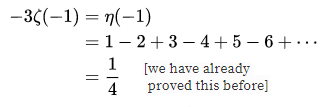[Note: We can also compute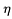(-1) by taking one-sided limit, but I skipped that to keep things simple.]

Thus, we proved the result in a more rigorous way. However, Ramanujan also developed his own formula to solve these type of infinite divergent series. This method is known as Ramanujan Summation. However, this is little more advanced and may not suit general readers. But, interested readers may check it here.

Ramanujan in his second letter to G. H. Hardy wrote:"Dear Sir, I am very much gratified on perusing your letter of the 8th February 1913. I was expecting a reply from you similar to the one which a Mathematics Professor at London wrote asking me to study carefully Bromwich's Infinite Series and not fall into the pitfalls of divergent series. … I told him that the sum of an infinite number of terms of the series: 1 + 2 + 3 + 4 + ⋯ = − 1/12 under my theory. If I tell you this you will at once point out to me the lunatic asylum as my goal. I dilate on this simply to convince you that you will not be able to follow my methods of proof if I indicate the lines on which I proceed in a single letter. …".......
Ramanujan, in his second letter to G. H. Hardy, dated 27 February 1913

Now, let's see how we can represent the series of infinite natural numbers visually. As we know that the formula for the sum of n infinite natural numbers is,

Sn =[Which is also the formula for partial sums.]

If we plot a graph for all the positive value we get from partial sums, it will look something like this:Here you can see the curve goes to infinity as n goes to infinity. So far so good. But what if we plot the graph for various values of 'n' even including the negative values? If we do that we'll get a graph like this: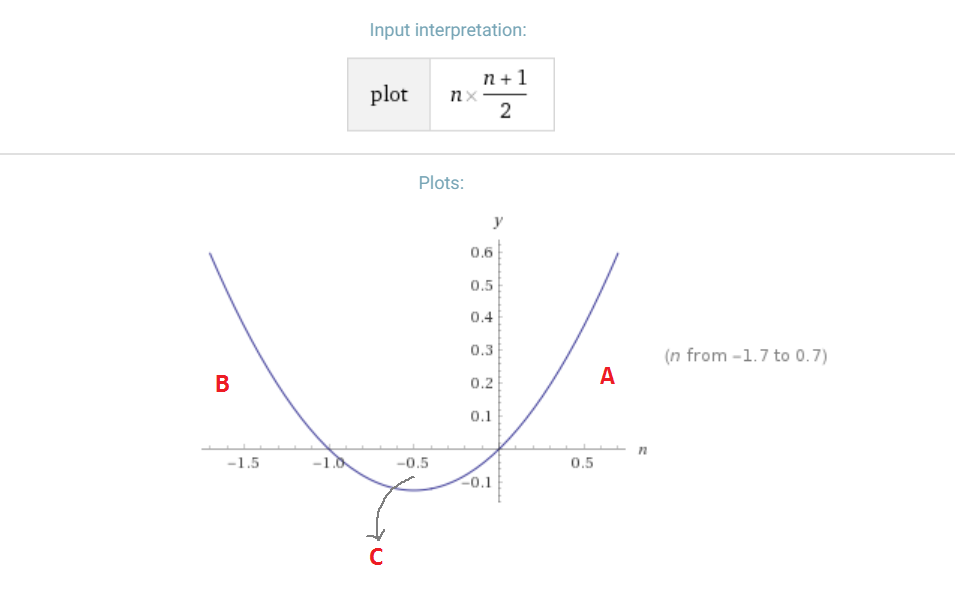We get three interesting areas (A, B and C) in the graph. Two are above the x-axis and one is below it. The two areas above the horizontal axis are actually infinite as the curve extends to infinity. But the area 'C', which is below the horizontal axis is the most interesting one. It is small and closed by the curve and the horizontal axis. So, it has a definite value. And if we use calculus to determine the area of the closed part 'C' we shall get 1/12 sq. units and as it is below the horizontal axis, it should be -1/12 sq. units:Really amazing, isn't it? The curve generated by the partial sums of the series 1 + 2 + 3 + 4 + · · · gives us a graph with a little closed region of area. And this is how the valueis associated with infinite series of natural numbers.

Now, I think you have understood the whole fact thatis a characteristic of the curve of 1 + 2 + 3 + 4 + · · · So, we can't apply this value everywhere. However, there are some special fields of study where we can use this result.

So, How is this Result Useful?String theory | Credits: nkrumahsteward

This result is very useful in many fields of Physics. For example, in the bosonic string theory, result is used to calculate the total energy of infinite number of quantum harmonic oscillations. And this fact is also used to tell that string theory is not consistent in dimensions other than 26.

The regularization of 1 + 2 + 3 + 4 + · · · is also helpful in computing the Casimir force for a scalar field in one dimension. The negative sign in -1/12 reflects the fact that the Casimir force is attractive.

This amazing result may also be in use in many other fields of quantum mechanics and many more in future.

The Conclusion

So, mathematics, which is very much logical, sometimes gives us weird results. Either we're doing something wrong or our universe is just like that. As I said in my previous post on Antimatter, sometimes math gives us some weird results which are against our intuition and physicists ignore such results as mathematical artefacts. But sometimes they may be real. I don't know the fortune of -1/12 calculated by analytical continuation of 1 + 2 + 3 + 4 + . . . But one thing is sure, it is helping a lot in many calculations in Physics. I am really impressed with the work of the genius young mathematician Srinivasa Ramanujan.

Writing this post was really an amazing experience. I love Math and I hope after reading this post you will also start liking it. This post turned out to be much longer than I expected. I also thought to divide it into two parts, but that would break the flow of thought and might create confusion. So, leaving it as it is. Yeah, in the last part (Riemann zeta function) it became little bit complicated for general readers, pardon me for that, however, I tried to keep rest of the post very much simple and lucid. I know, I know, no one gets a Nobel Prize for Math. But I hope this post will be liked and accepted by all the enthusiastic mathematicians out there. Whether I write more similar posts or not depends on the response I get on this post. So, if you have any question or doubt or if you have read the post and liked it, don't forget to leave a comment.

Thanks. :)

Image Source :

All the non cited images are either taken from Pixabay.com, Pexels.com, or Wikipedia.com and are available for Reuse under Creative Commons Licenses or created by me.

Graphs are created using : www.wolframalpha.com

The SteemSTEM Coin Logo used in the title image is created by @mindhacks. A special thanks to him. :)

ReferencesFor more discussion on this topic (or anything related to science), please join us on steemSTEM. SteemSTEM is a community driven project which seeks to promote well written/informative Science Technology Engineering and Mathematics postings on Steemit. More information can be found on the @steemstem blog.

Want more stuff like this directly in your feed:

Also catch me on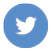--- thinknitesh.com ---

Sort Order:
·  2 years ago

Infinity is a very hard topic to grasp, but very fun to play around with. Looking forward to your next post

·  2 years ago

Yeah, the incomprehensibility of the infinity makes it more interesting to deal with it. :D

·  2 years ago

3Blue1Brown has a very nice video on the subject as well. It might not be as detailed as your article but then I would not have understood your article if I had not seen the video first. so I leave it here:

·  2 years ago

Oh, thanks for sharing the video here. Will be helpful to the readers. :)

·  2 years ago (edited)

Srinivasa Ramanujan was an excellent treasure for Mathematics. If he had been in today's world with all these technological advancements, mathematics would have gone to a different level. Lots of pages from his notebook are still a mystery. If he had taken care of his health, he would have been able to give lot more than what he has given to us. Maybe as you said, that's how the universe works. 😊

·  2 years ago

Yeah, you're right. Ramanujan was an unprecedented talent. If he could have lived more, he could have given a lot more of mathematics.

·  2 years ago

being a masters physics student i can understand the effort and time you have given for this post.Are you a mathematician or physicist?

·  2 years ago

Oh. Thanks. I am thinker. I love to play around with math and science to see the world in a more comprehensive way.

BTW, there is an app called musical.ly. Is your name inspired by that? :p

(Initially I thought yours is official account of musical.ly. LOL)

·  2 years ago

Congratulations @nitesh9! You have completed some achievement on Steemit and have been rewarded with new badge(s) :Award for the number of comments

Click on any badge to view your own Board of Honor on SteemitBoard.

If you no longer want to receive notifications, reply to this comment with the word STOP

Upvote this notification to help all Steemit users. Learn why here!

·  last year

Congratulations @nitesh9! You have received a personal award!1 Year on Steemit
Click on the badge to view your Board of Honor.

Do not miss the last post from @steemitboard!

Participate in the SteemitBoard World Cup Contest!
Collect World Cup badges and win free SBD
Support the Gold Sponsors of the contest: @good-karma and @lukestokes

Do you like SteemitBoard's project? Then Vote for its witness and get one more award!

·  last year

Congratulations @nitesh9! You have completed the following achievement on the Steem blockchain and have been rewarded with new badge(s) :Award for the number of upvotes

Click on the badge to view your Board of Honor.
If you no longer want to receive notifications, reply to this comment with the word STOP

Support SteemitBoard's project! Vote for its witness and get one more award!

·  4 months ago

Congratulations @nitesh9! You received a personal award!Happy Birthday! - You are on the Steem blockchain for 2 years!

You can view your badges on your Steem Board and compare to others on the Steem Ranking

Do not miss the last post from @steemitboard:The Steem community has lost an epic member! Farewell @woflhart!SteemitBoard - Witness UpdateDo not miss the coming Rocky Mountain Steem Meetup and get a new community badge!